# Colorful Image Colorization Trained onImageNet Competition Data

Colorize a grayscale image

Released in 2016, this model recasts image colorization into a classification problem by dividing the AB color space into 313 bins. The final color for each pixel is picked by performing an ad hoc modified mean of its probability distribution over the bins. The resulting method produces vibrant and realistic colorizations.

Number of layers: 58 | Parameter count: 32,241,005 | Trained size: 129 MB |

## Examples

### Resource retrieval

Get the pre-trained net:

 In:=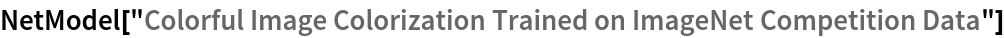Out=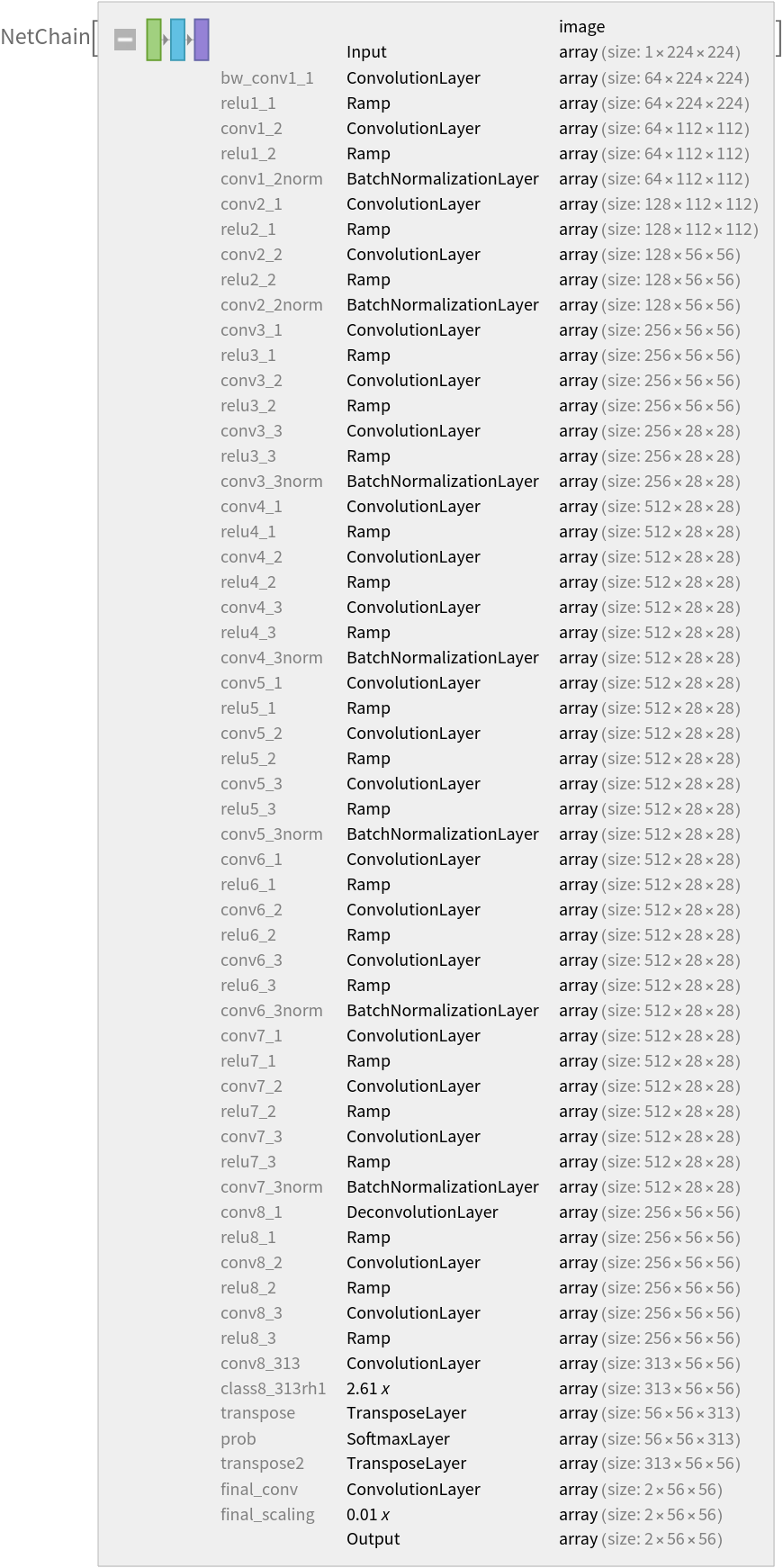### Evaluation function

This net takes a grayscale image as input and outputs the A and B channels in the LAB color space. It needs an evaluation function to merge its output with the luminance of the input:

 In:=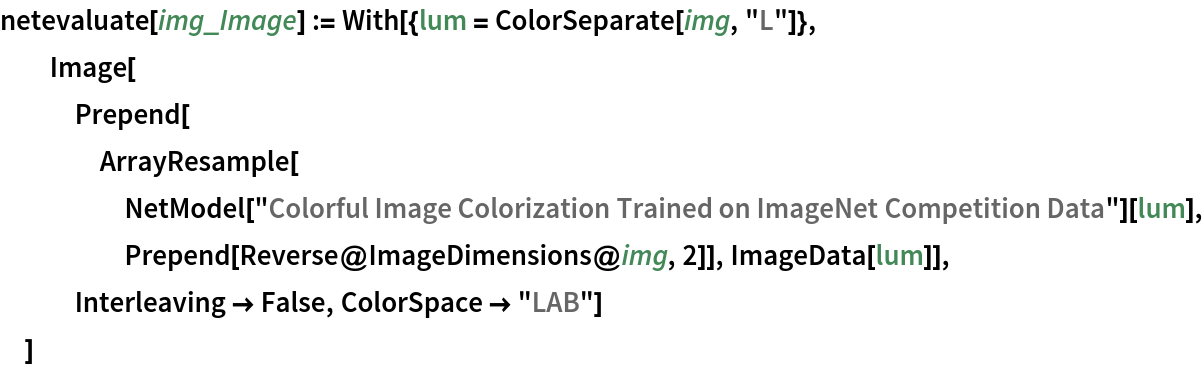### Basic usage

Colorize a grayscale image using the evaluation function:

 In:=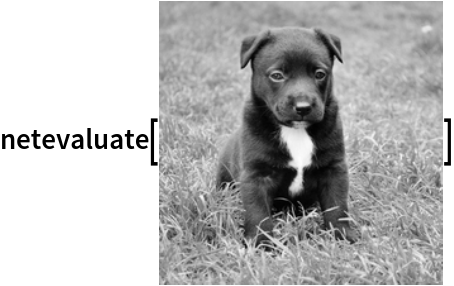Out=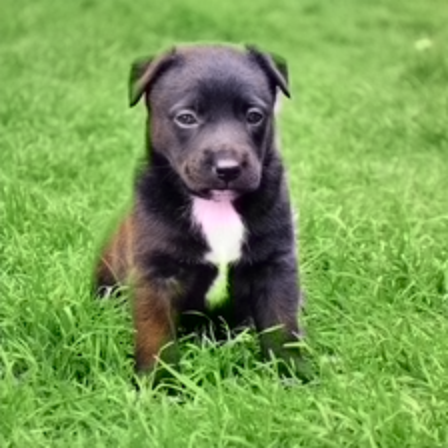### Performance evaluation

Get a color image:

 In:=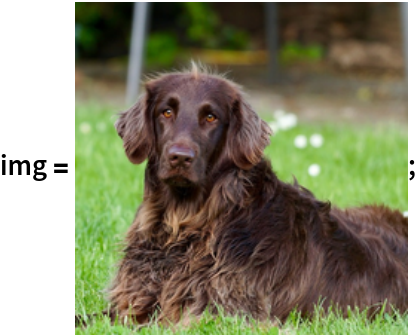Compare the colorization performed by the net with the ground truth:

 In:=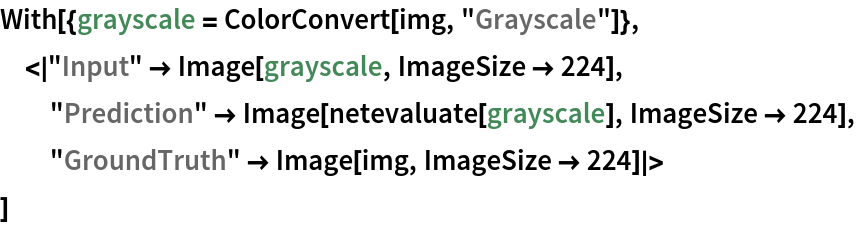Out=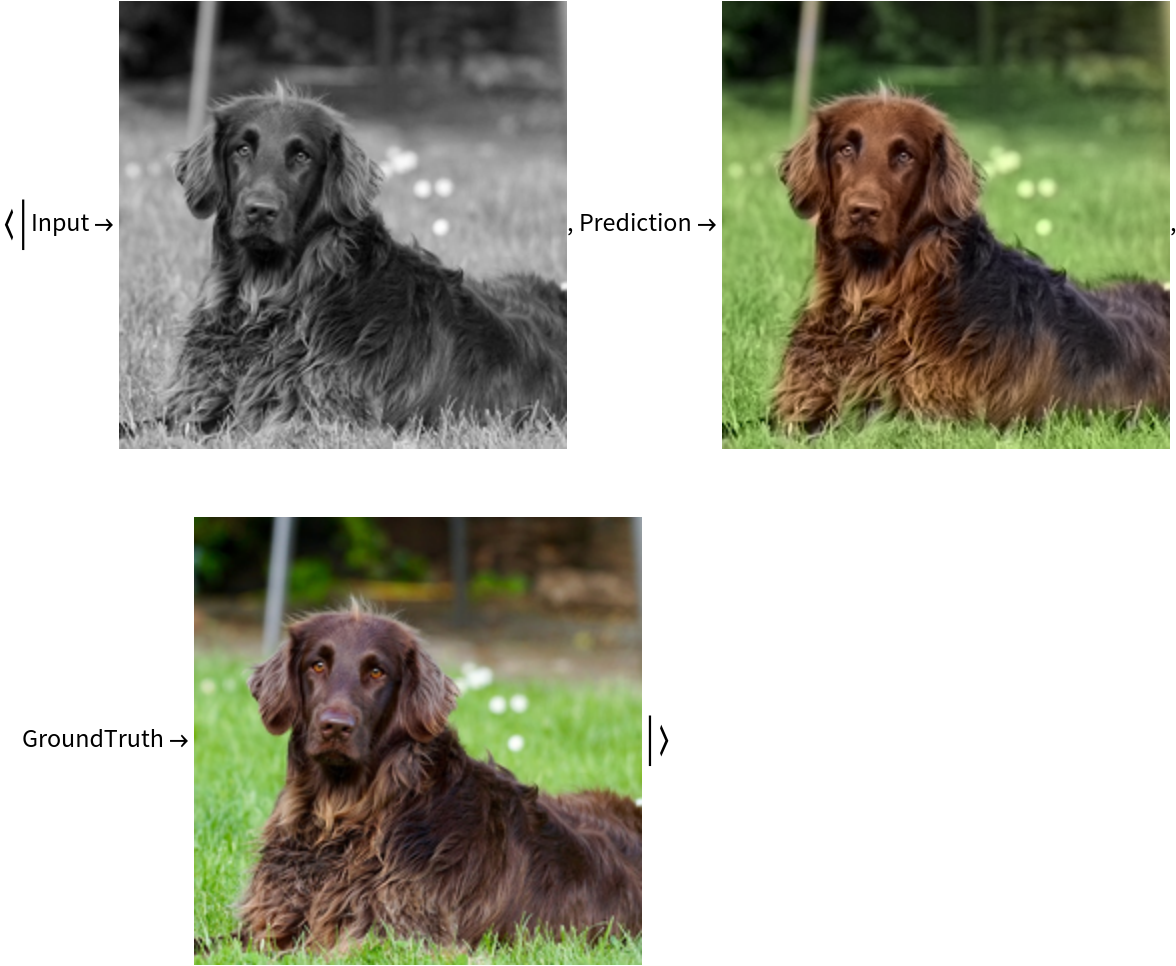### Net information

Inspect the number of parameters of all arrays in the net:

 In:=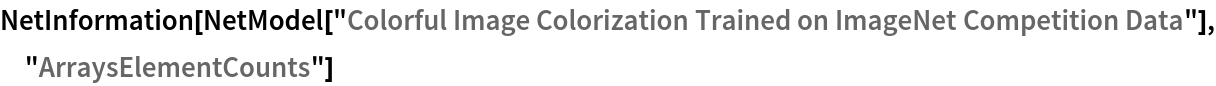Out=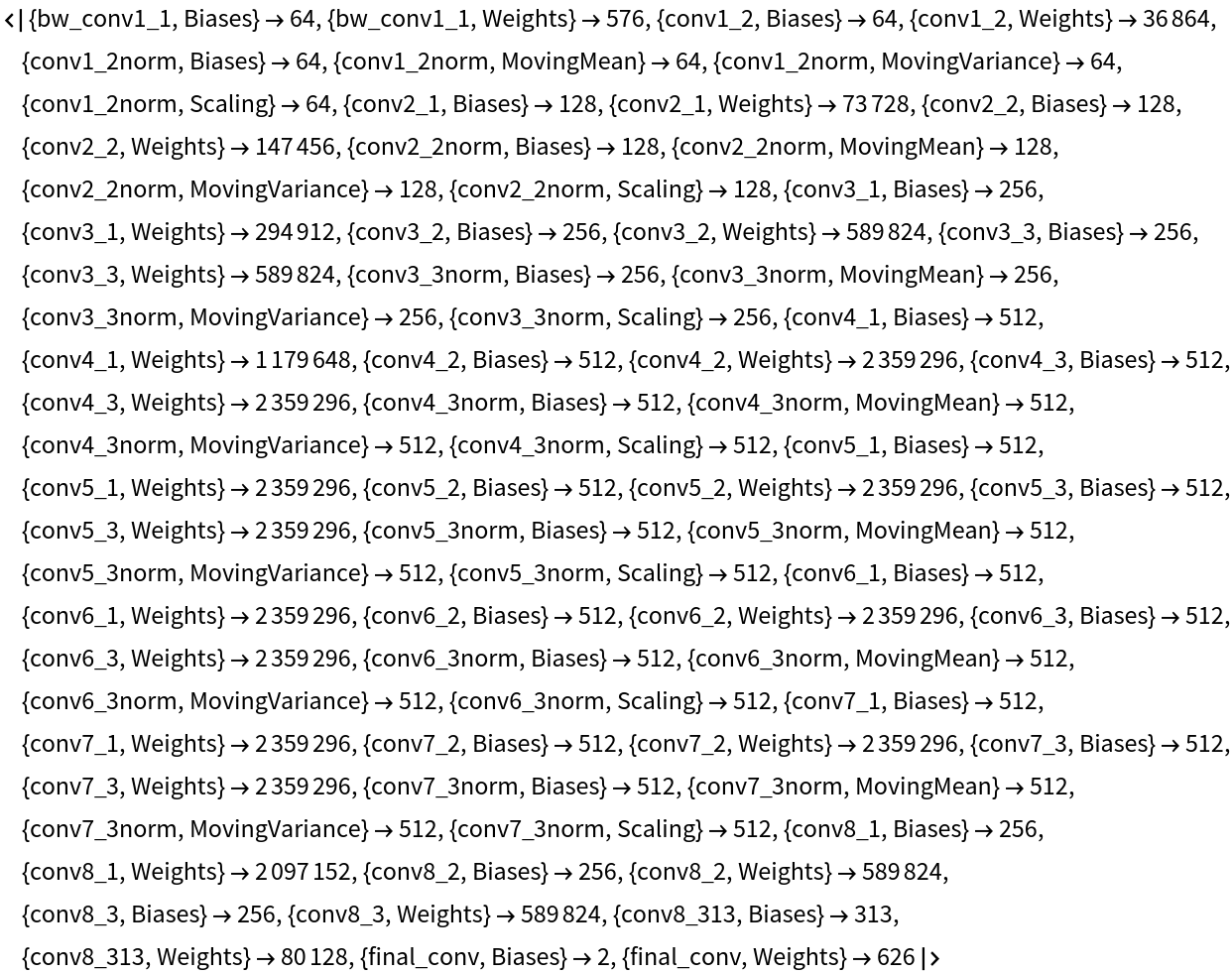Obtain the total number of parameters:

 In:=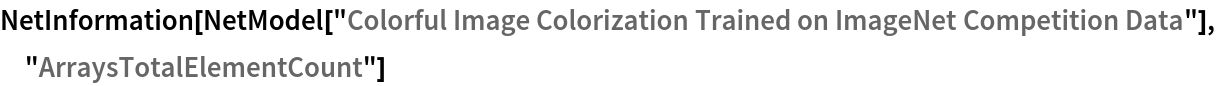Out=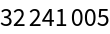Obtain the layer type counts:

 In:=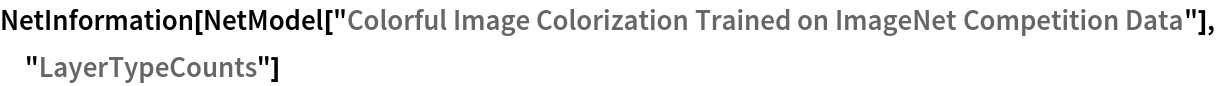Out=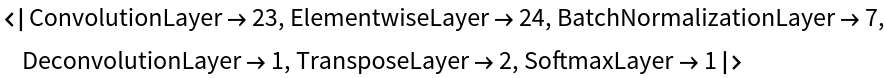Display the summary graphic:

 In:=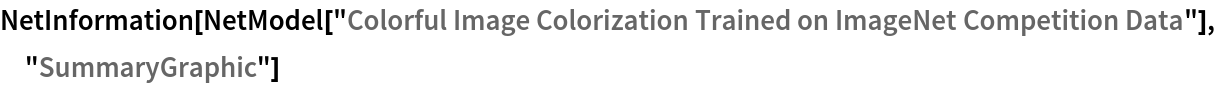Out=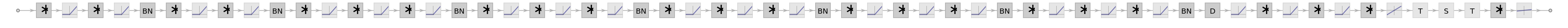### Export to MXNet

Export the net into a format that can be opened in MXNet:

 In:=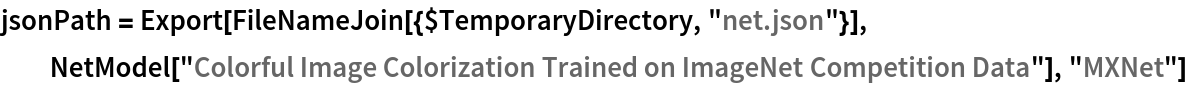Out=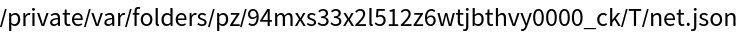Export also creates a net.params file containing parameters:

 In:=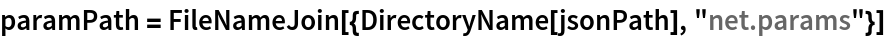Out=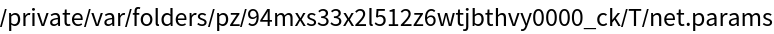Get the size of the parameter file:

 In:=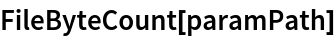Out=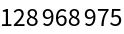The size is similar to the byte count of the resource object:

 In:=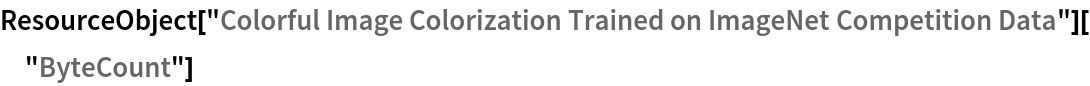Out=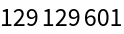Represent the MXNet net as a graph:

 In:=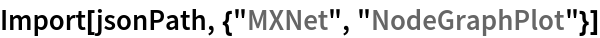Out=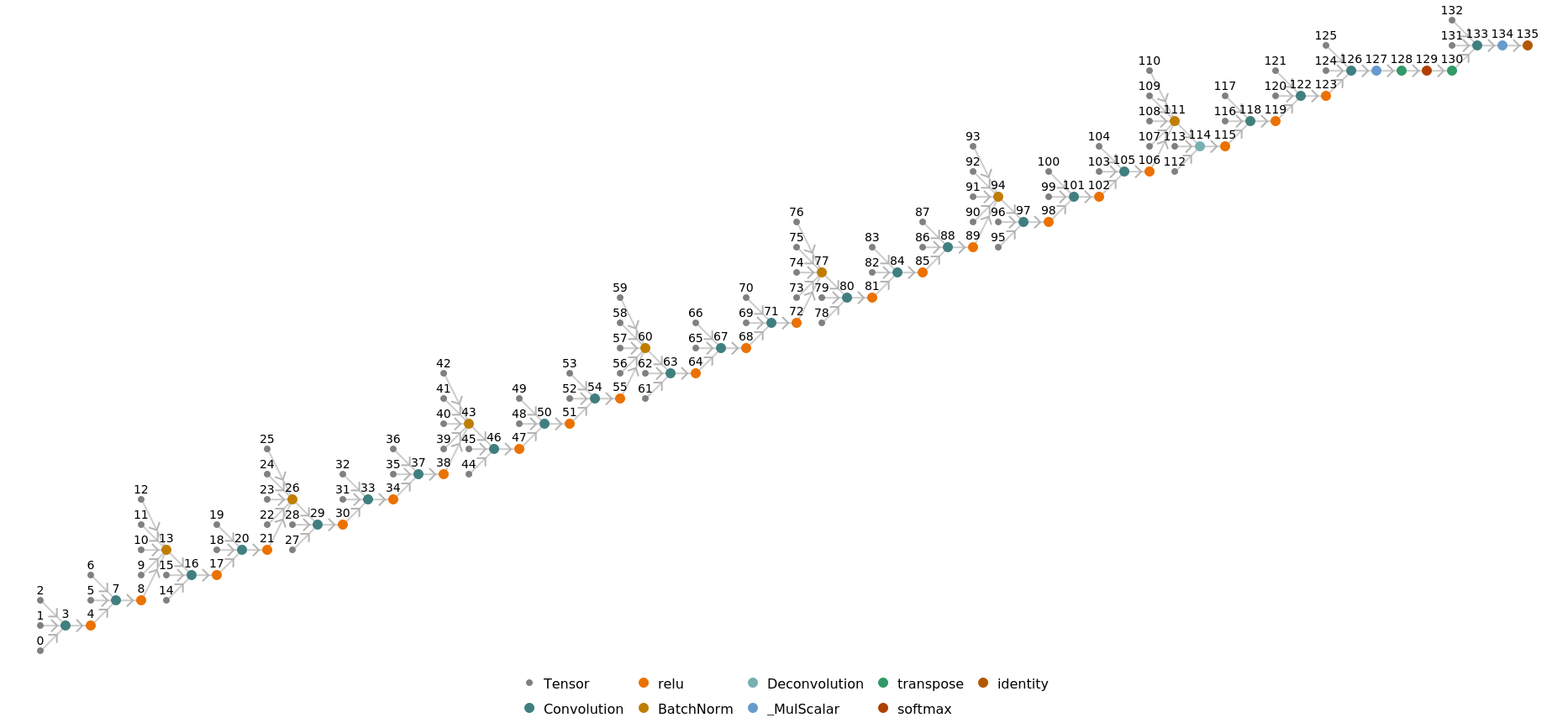## Requirements

Wolfram Language 11.3 (March 2018) or above